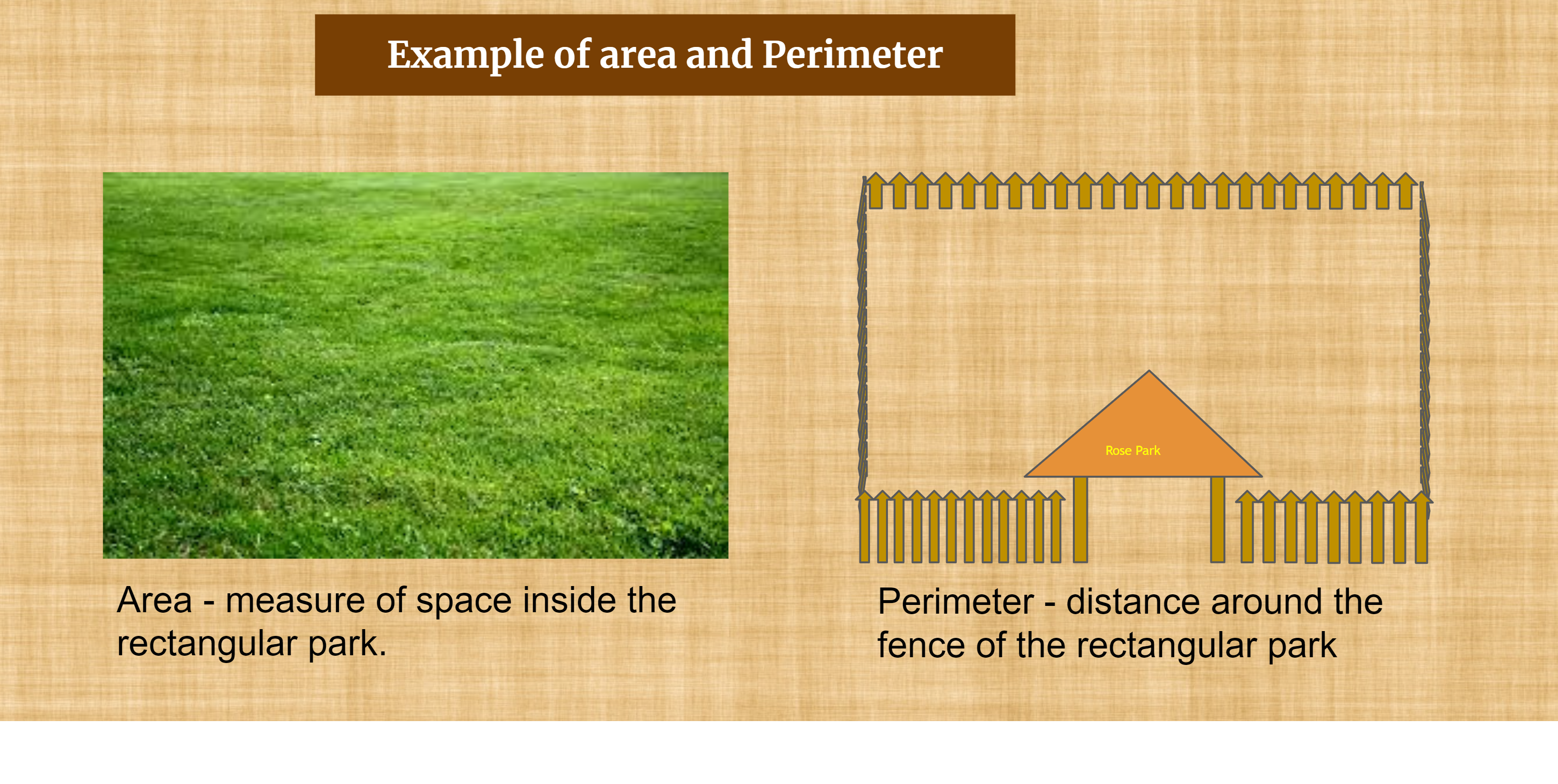# Area and Perimeter

``````

Dear Volunteer Teacher,

Kindly teach the bridge concept of Area and Perimeter prior to teaching the related textbook chapters.
Thank you.

## Course Content

• Defintions of area and perimeter
• Area and perimeter of square and rectangle.
• Situation Area and perimeter of triangles - Situation
• Formula for area and perimater for Square, Rectangle, Triangle and Parallelogram

## Learning Outcomes

Learning outcomes has been listed below which will serve as your guide for what students need to learn.

SWBAT:

• understand the concept of Area and Perimeter
• calclulate the area and perimeter for a Square, Rectangle and Triangle and parallelogram

## PPT Deck

To make the lesson come alive, please find below PPT deck